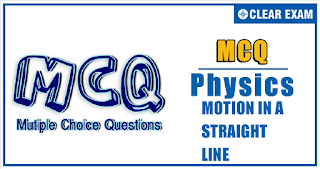## Physics Quiz-7

If an object changes its position with respect to its surroundings with time, then it is called in motion. It is a change in the position of an object over time. Motion in a straight line is nothing but linear motion. As the name suggests, it’s in a particular straight line, thus it can be said that it uses only one dimension.

Q1. A juggler keeps on moving four balls in air throwing the balls after regular intervals. When one ball leaves his hand (speed =20 ms^(-1)) the position of other balls (height in meter) will be (take g =10 ms^(-2)).
•  10,20,10
•  15,20,15
•  5,15,20
•  5,10,20

Solution
Time taken by the same ball to return to the hands of the juggler is 2u/g=(2×20)/10=4 s. So he is throwing the balls after 1 s each. Let at some instant he throws ball number 4. Before 1 s of throwing it, he throws ball 3. So the height of ball 3 is
h_3=20×1-1/2 10(1)^2=15 m
Before 2 s, he throws ball 2. So the height of ball 2 is
h_2=20×2-1/2 10(2)^2=20 m
Before 3 s, he throws ball 1. So the height of ball 1 is
h_1=20×3-1/2 10(3)^2=15 m

Q2.The numerical value of the ratio of average velocity to average speed is.
•  Always less than one
•  Always equal  to one
•  Always more than one
•  Equal to or less than one
Solution
We know that average velocity =Displacement/time and average speed =Distance/time
Since displacement can be less than or equal to distance, average velocity can be less than or equal to average speed.

Q3. A ball is dropped into a well in which the water level is at a depth h below the top. If the speed of sound is c, then the time after which the splash is heard will be given by.
Solution
Time of fall =√(2h/g)
Time taken by the sound to come out =h/c
Total time =√(2h/g)+h/c=h[√(2/gh)+1/c]

Q4. The velocity time graph of a body is shown in Fig. It indicates that

•  At B force is zero
•  At B there is a force but towards motion
•  At B there is a force which opposes motion
•  None of the above is true
Solution
Near B velocity decreases with time. Hence, there is retardation or there is opposing force acting on the body.

Q5.An object is thrown up vertically. The velocity-time graph for the motion of the particle is
Solution
At t=0, velocity is positive and maximum. As the particle goes up, velocity decreases and becomes zero at the highest point. When the particle starts coming down, velocity increases in the negative direction.

Q6.
 The variation of velocity of a particle moving along a straight line is shown in Fig. The distance travelled by the particle in 12 s is•  37.5 m
•  32.5 m
•  35.0 m
•  None of these
Solution
Area from 0 to 10 s =1/2 [10+4]5=35 m
Area from 10 to 12 s =1/2×2×(-2.5)=-2.5 m
Distance travelled =35+2.5=37.5 m

Q7.
Two trains, one travelling at  and other at , are heading towards one another along a straight track. Both the drivers apply brakes simultaneously when they are 500 m apart. If each train has a retardation of , the separation after they stop is
•  192.5 m
•  225.5 m
•  187.5 m
•  155.5 m
Solution
Distance travelled by first train
s_1=u^2/2a=(15)^2/(2×1)=112.5 m
Distance travelled by second train
s_2=(20)^2/(2×1)=200 m
Total distance travelled =112.5+200=312.5 m
Distance of separation =500-312.5=187.5 m

Q8. A parachutist drops first freely from an airplane for 10 s and then his parachute opens out. Now he descends with a net retardation of 2.5 ms^(-2). If he bails out of the plane at a height of 2495 m and g =10 ms^(-2), his velocity on reaching the ground will be
•  5 ms^(-1)
•  10 ms^(-1)
•  15 ms^(-1)
•  20 ms^(-1)
Solution
The velocity v acquired by the parachutist after 10 s:
v=u+gt=0+10×10=100 ms^(-1)
Then, s_1=ut+1/2 gt^2=0+1/2×10×10^2=500 m
The distance travelled by the parachutist under retardation is
s_2=2495-500=1995 m
Let v_g be the velocity on reaching the ground. Then v_g^2-v^2=2as_2
or v_g^2-(100)^2=2×(-2.5)×1995 or v_g=5 ms^(-1)

Q9.
 Two cars are moving in same direction with speed of 30 kmh^(-1). They are separated by a distance of 5 km. What is the speed of a car moving in opposite direction if it meets the two cars at an interval of 4 min?

•  60 km/h
•  5 km/h
•  30 km/h
•  45 km/h

• Solution
t=S_rel/v_rel  ⇒  4/60=5/(30+v_2 )  ⇒  v_2=45 kmh^(-1)
Q10. A stone is dropped from the 25th floor of a multistoried building and it reaches the ground in 5 s. In the first second, it passes through how many floors of the building? (g =10 ms^(-2))
•  1
•  2
•  3
•  None of these
Solution
Suppose h be the height of each floor, then
25h=0+1/2×10×t^2=1/2×10×5^2 or h=5 m
In first second, let the stone passes through n floor. So
n×5=1/2×10×(1)^2 or n=1#### Written by: AUTHORNAME

AUTHORDESCRIPTION## Want to know more

Please fill in the details below:

## Latest NEET Articles\$type=three\$c=3\$author=hide\$comment=hide\$rm=hide\$date=hide\$snippet=hide

Name

ltr
item
BEST NEET COACHING CENTER | BEST IIT JEE COACHING INSTITUTE | BEST NEET & IIT JEE COACHING: Motion In a Straight Line-Quiz-7
Motion In a Straight Line-Quiz-7
https://1.bp.blogspot.com/-_KLB3j4_t04/YN796ZrnaJI/AAAAAAAAQV0/6p5Bk2YWE0Qp2o09-L6Sq_jMUsUSTTwJwCLcBGAsYHQ/s320/QUIZ%2BBACK.png
https://1.bp.blogspot.com/-_KLB3j4_t04/YN796ZrnaJI/AAAAAAAAQV0/6p5Bk2YWE0Qp2o09-L6Sq_jMUsUSTTwJwCLcBGAsYHQ/s72-c/QUIZ%2BBACK.png
BEST NEET COACHING CENTER | BEST IIT JEE COACHING INSTITUTE | BEST NEET & IIT JEE COACHING
https://www.cleariitmedical.com/2021/07/motion-in-straight-line-quiz-7.html
https://www.cleariitmedical.com/
https://www.cleariitmedical.com/
https://www.cleariitmedical.com/2021/07/motion-in-straight-line-quiz-7.html
true
7783647550433378923
UTF-8

STAY CONNECTED